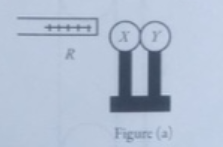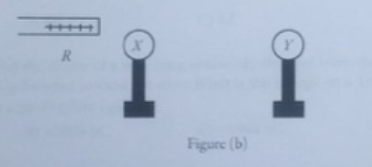# Problem: 1)Two large, flat, horizontally oriented plates are parallel to each other, a distance d apart. Half way between the two plates the electric field has magnitude E. If the separation of the plates is reduced to d/2 what is the magnitude of the electric field half way between the plates?A) 0B) E/2 C) 2E D) 4E E) E 2) X and Y are two uncharged metal spheres on insulating stands, and are in contact with each. A positively charged rod R is brought close to X as shown in Figure (a). Sphere Y is now moved away from X, as in Figure (b). What are the final charge states of X and Y? A) X is neutral and Y is positive. B) X is negative and Y is positive. C) Both X and Y are neutral.D) X is positive and Y is neutral. E) Both X and Y are negative. 3) A piece of plastic has a net charge of +2.00 μC. How many more protons than electrons does this piece of plastic have? (e = 1.60 × 10-19 C). A) 1.25 × 1019 B) 2.50 × 1019 C) 1.25 × 1013D) 2.50 × 1013

###### FREE Expert Solution

Gauss's law:

$\overline{)\begin{array}{rcl}{\mathbf{\varphi }}_{\mathbf{E}}& {\mathbf{=}}& \mathbf{\oint }\mathbf{E}\mathbf{·}\mathbf{d}\mathbf{A}\\ & {\mathbf{=}}& \frac{{\mathbf{Q}}_{\mathbf{e}\mathbf{n}\mathbf{c}}}{{\mathbf{\epsilon }}_{\mathbf{0}}}\end{array}}$

90% (260 ratings)###### Problem Details

1)Two large, flat, horizontally oriented plates are parallel to each other, a distance d apart. Half way between the two plates the electric field has magnitude E. If the separation of the plates is reduced to d/2 what is the magnitude of the electric field half way between the plates?

A) 0

B) E/2

C) 2E

D) 4E

E) E

2) X and Y are two uncharged metal spheres on insulating stands, and are in contact with each. A positively charged rod R is brought close to X as shown in Figure (a).Sphere Y is now moved away from X, as in Figure (b).What are the final charge states of X and Y?

A) X is neutral and Y is positive.

B) X is negative and Y is positive.

C) Both X and Y are neutral.

D) X is positive and Y is neutral.

E) Both X and Y are negative.

3) A piece of plastic has a net charge of +2.00 μC. How many more protons than electrons does this piece of plastic have? (e = 1.60 × 10-19 C).

A) 1.25 × 1019

B) 2.50 × 1019

C) 1.25 × 1013

D) 2.50 × 1013